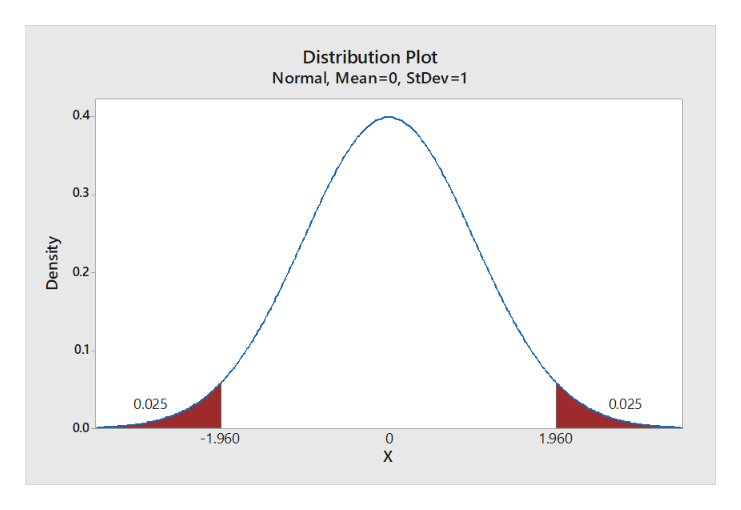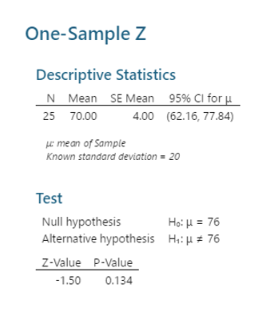# Manhattan College is evaluating a new English composition course for freshman.  A random sample of n = 25 freshman is obtained and the students are placed in the course during their first semester.  One year later, a writing sample is obtained for each student and the writing samples are graded using a standardized evaluation technique. The average score for the sample is M = 76.  For the general population of college students, writing scores form a normal distribution with a mean of μ = 70 and a standard deviation of σ = 20.What are the independent and dependent variables?Using symbols, state the hypotheses for the type of test you indicated in part b. (H0and H1)Sketch the appropriate distribution and locate the critical region for α = .05 for the type of test you indicated in part b.Test the researcher’s hypothesis using the appropriate statistic.What decision should be made about the null hypothesis? Be sure to use the correct language when answering this question.Compute Cohen’s dto measure the size of the effect. Is it a small, medium or large effect?Write a sentence describing the outcome of the hypothesis test and the measure of effect size as it would appear in a research report.

Question
2 views

Manhattan College is evaluating a new English composition course for freshman.  A random sample of n = 25 freshman is obtained and the students are placed in the course during their first semester.  One year later, a writing sample is obtained for each student and the writing samples are graded using a standardized evaluation technique. The average score for the sample is M = 76.  For the general population of college students, writing scores form a normal distribution with a mean of μ = 70 and a standard deviation of σ = 20.

1. What are the independent and dependent variables?
2. Using symbols, state the hypotheses for the type of test you indicated in part b. (H0and H1)
3. Sketch the appropriate distribution and locate the critical region for α = .05 for the type of test you indicated in part b.
4. Test the researcher’s hypothesis using the appropriate statistic.
5. What decision should be made about the null hypothesis? Be sure to use the correct language when answering this question.
6. Compute Cohen’s dto measure the size of the effect. Is it a small, medium or large effect?
7. Write a sentence describing the outcome of the hypothesis test and the measure of effect size as it would appear in a research report.

check_circle

Step 1

Hey, since there are multiple subparts posted, we will answer first four question. If you want any specific question to be answered then please submit that question only or specify the question number in your message.

(1)

Here, the sample is independent. Because 25 freshman are selected and the students are placed in the course during their first semester.  A writing sample is obtained for each student and the writing samples are graded using a standardized evaluation technique.

(2)

The hypotheses are given below:

Null hypothesis:

H0: μ = 70

Alternative hypothesis:

H1: μ ≠70

(3)

Step-by-step procedure to obtain the critical value using MINITAB:

• Choose Graph > Probability Distribution Plot choose View Probability > OK.
• From Distribution, choose ‘Normal’ distribution.
• Choose Probability and Both Tail for the region of the curve to shade.
• Enter the Probability value as 05.
• Click OK.

Output using the MINITAB software is given below:help_outlineImage TranscriptioncloseDistribution Plot Normal, Mean-0, StDev=1 0.4 0.3 0.2 0.1 0.025 0.025 0.0 -1.960 1.960 0 Density fullscreen
Step 2

From the output, the critical value is ±1.96 and the shaded region is the rejection region.

4.

Step by step procedure to obtain the test statistic using MINITAB software is given below

• Choose Stat > Basic Statistics > 1-Sample Z.
• In Summarized data, enter the sample size as 25 and mean as 76.
• In Standard deviation, enter 20.
• Check Options, enter Confidence level as 95.
• In Perform Hypothesis test, enter 70 Under Hypothesized mean.
• Choo...help_outlineImage TranscriptioncloseOne-Sample Z Descriptive Statistics N Mean SE Mean 95% Cl for 4.00 (62.16, 77.84) 25 70.00 mean of Sample Known standard deviation 20 Test Hs μ- 76 Null hypothesis Alternative hypothesis H:76 Z-Value P-Value 0.134 -1.50 fullscreen

### Want to see the full answer?

See Solution

#### Want to see this answer and more?

Solutions are written by subject experts who are available 24/7. Questions are typically answered within 1 hour.*

See Solution
*Response times may vary by subject and question.
Tagged in

### Hypothesis Testing